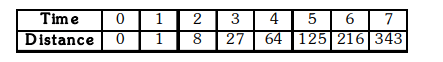# The following is the distance-time table of an object in motionQuestion.
The following is the distance-time table of an object in motion(a) What conclusion can you draw about the acceleration ? Is it constant, increasing, decreasing or zero ?
(b) What do you infer about the force acting on the object?

Solution:

(a) A careful observation of the distance-time table shows that $\mathrm{s} \propto \mathrm{t}^{3}$.

It is known that, in uniform motion $\mathrm{s} \propto \mathrm{t}$, and for motion with uniform acceleration $\mathrm{s} \propto \mathrm{t}^{2}$.

In the present case, $\mathrm{s} \propto \mathrm{t}^{3}$. Therefore, we conclude in this case that acceleration must be

increasing with time.

(b) As $F=m a$, therefore, $F \propto$ a. Hence, the force must also be increasing with time.